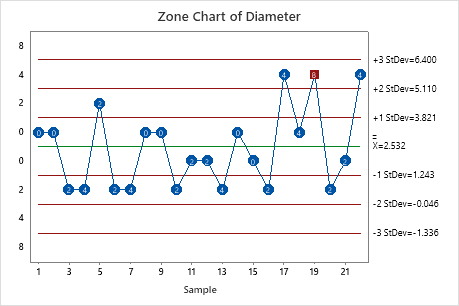# Interpret the key results for Zone Chart

Complete the following steps to interpret a zone chart. Key output includes the zone chart and test results.

## Step 1: Determine whether the process mean is stable

The zone chart plots the cumulative scores based on zones at 1, 2, and 3 standard deviations from the center line. The center line is the overall average of the individual observations or subgroup averages. Each plotted point is assigned a weight based on how far that point is from the center line.

The zone chart consists of four zones that have different weights:
• Zone 1 is between the center line and 1σ and has a weight of 0
• Zone 2 is between 1σ and 2σ and has a weight of 2
• Zone 3 is between 2σ and 3σ and has a weight of 4
• Zone 4 is beyond 3σ and has a weight of 8

The cumulative score is a sum of the weights of the plotted points. Each time the points cross the center line, the cumulative score is reset to zero. Each cumulative score helps to determine whether the process is in control. When the cumulative score is greater than or equal to 8, the process is out of control.In these results, subgroup 19 has a cumulative score of 8. Investigate this subgroup.

## Step 2: Identify which points failed each test

Each time the points cross the center line on a zone chart, the cumulative score is set to zero. Each cumulative score helps determine whether or not the process is in control. When the cumulative score is greater than or equal to 8, the process has a nonrandom pattern in the data that may be a result of special-cause variation. Out-of-control points are reported in the output.

By using this site you agree to the use of cookies for analytics and personalized content.  Read our policy